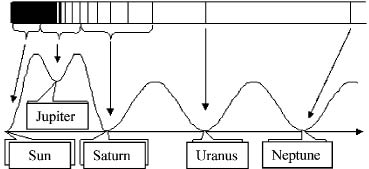# Scientific journal European Journal of Natural History ISSN 2073-4972

### MAXWELL THEORY DESCRIBES SOLAR SYSTEM

An idea of oscillator exists in quantum mechanics. Let us show, that the planet system really is a linked system of pendulums. A criterion of the platen orbits and the whole planet system steadiness can be the condition:

Tj/Tij = m + 1, (1)

where m = 1,2... is a whole positive number. For planets with the circulation period Ti and Tj (Ti < Tj) the meeting period Tij equals

1/Tij = 1/Ti + 1/Tj, or Tij = (Ti∙Tj)/(Ti + Tj) (2)

If we substitute this equation into the initial one, we will obtain the oscillator equation:

Ti = Tj/m. (3)

As Tj we will choose the period of the sun circulation around the system masses centre Tj = Tc and we will calculate m = Tc/Ti (table 1). Here Ti will be the Planets-Giants´ circulation period.

Table 1

The sun controls planets

 object Sun Jupiter Saturn Uranus Neptune pPluto Ti, years 179,77 11,86 29,45 84,01 164,8 247,7 Tij, years - 11,12 25,29 57,15 85,75 103,8 m - 15,07 6,07 2,13 1,09 0,72

Astronomers already refer Pluto to planets and this is confirmed by the fractional index m ≈ 3/4. For other planets this condition is accurately satisfied.

In table 1 index m runs through the values out of sequence, and that gives us grounds to suppose the final number of levels in system K = C/Vg = 22351 (this constant is responsible for the Universe structure). Here C is the light speed, Vg = (GN-K∙GK)½ is the graviton speed, GN-K is the constant of Newton-Cavendish, GK is the «magnet» gravity constant, M0 is the sun mass. In this case planets Neptune and Uranus are represented by one oscillator level each, and Saturn, Jupiter, and Sun level number is defined by the number of oscillator levels above each Sun gravity wave superposition holes - the graphic analogue of Schredinger equation (Figure and
table 2). The Sun main gravity wave length is λ0 = M0/GK, and period T0 = 2πλ0/Vg = 10,95 years. The sun gravity potential is represented by the sum of two waves. The main wave with length equals λ0 and is in same condition as wave with length 2λ0 with three condition of the «spin» projections number. «Spin» is also a quantum analogue.

Table 2

The results of the Planets-Giants´ mass calculation

 Planet Observed mass, g Calculated mass, g Number of levels Sun 1,99∙1033 - 22321 Jupiter 1,90∙1030 1,96∙1030 22 Saturn 5,68∙1029 5,34∙1029 6 Uranus 8,7∙1028 8,91∙1028 1 Neptune 10,3∙1028 8,91∙1028 1The mass of one oscillator level (gravity level) in system MUr equals:

MUr = M/K = 8,91∙1028 г,

here M is the Sun system mass

M ≈ M0 = 1,99∙1033 g. (4)

The observed Uranus and Neptune masses (МU = 8,7∙1028 g and МN = 10,3∙1028 g) corresponds to the oscillator level mass well. As all oscillator levels are identical, the other planet masses (and Sun) are defined by the number of oscillator levels above the corresponding gravity wave «hole». To put the obtained oscillator over the wave superposition the time is recalculated into the distance according to the Kepler law. The solar system has two energetic conditions. Jupiter is in the first condition, and Saturn, Uranus, and Neptune are in the second. The position of the Sun gravity potential is showed by Figure.

Therefore, the orbit radiuses, movement speeds, equator inclinations and masses of Planets-Giants are predefined by the Sun mass in the same way as the core charge defines the structure of atom electric cover.

Terrestrial planets were formed in the areas of the main wave interference (ℓ is a whole number):

R = λ0/(2∙ℓ + 1) (5)

If we carry on the quantum mechanics analogy, the «gravity radiation constant» k in a similar way with the Plank constant and «spin» of planets in this index units will look like:

k = p∙λ0

or

k = M∙Vg∙λ0 = M2∙(GN-K∙GK)½/GK = = M2∙(GN-K/GK)½ (6)

and

Skg = (k/2π)∙m = (M2/2π)∙(GN-K/GK)½∙m,

where m is the gravity «spin».

References

1. Kurkov A.A. Personal Internet - magazine. Registered on 02.06.2007 [digital source] / Science - News: site: URL: http://zaza149.inauka.ru/ (request date 13.01.2011).

The work is submitted to the International Scientific Conference «Priorities for Science, technology and engineering», Italy (Rome-Florence), 10-17 April 2011, came to the editorial office on 08.02.2011.